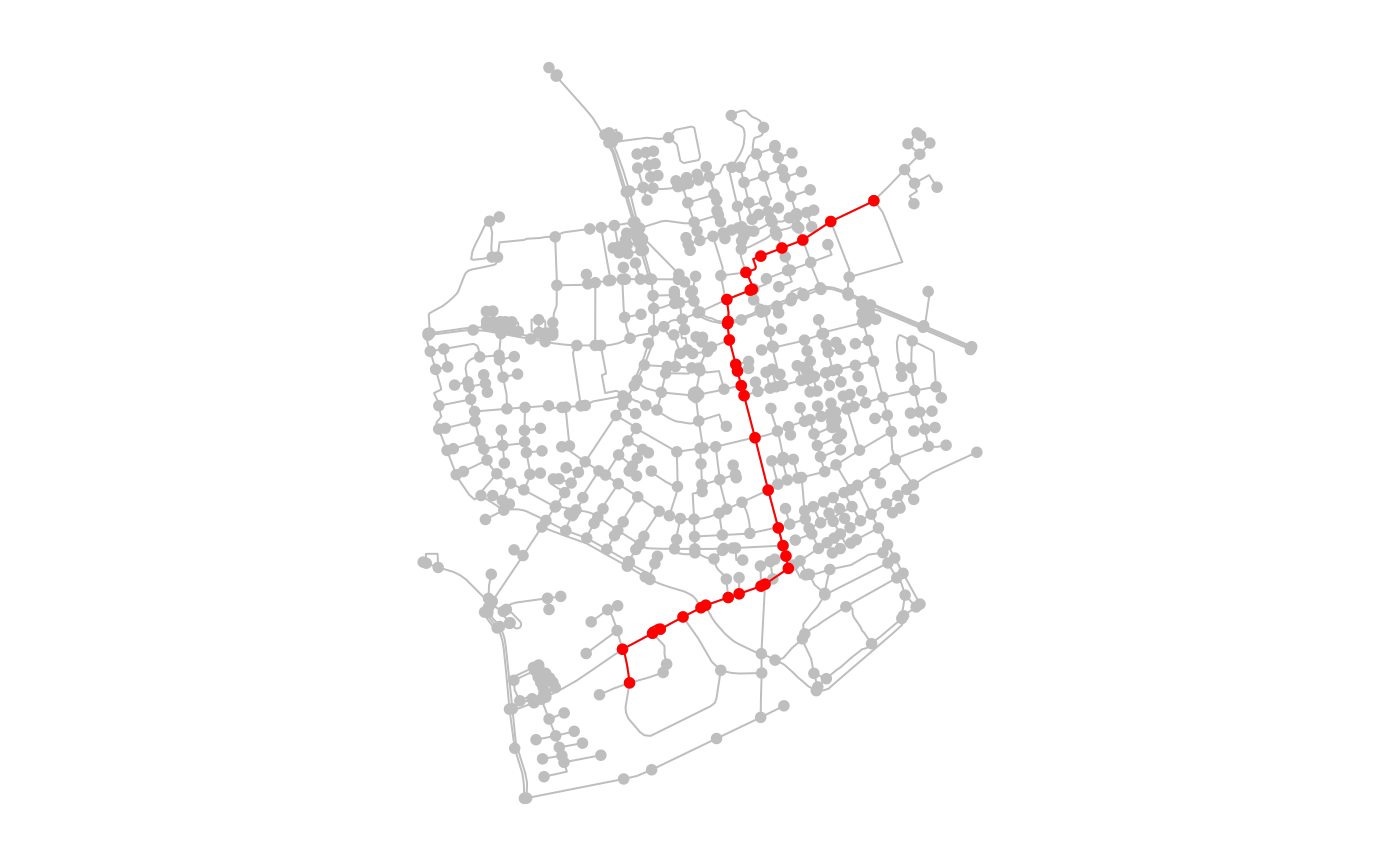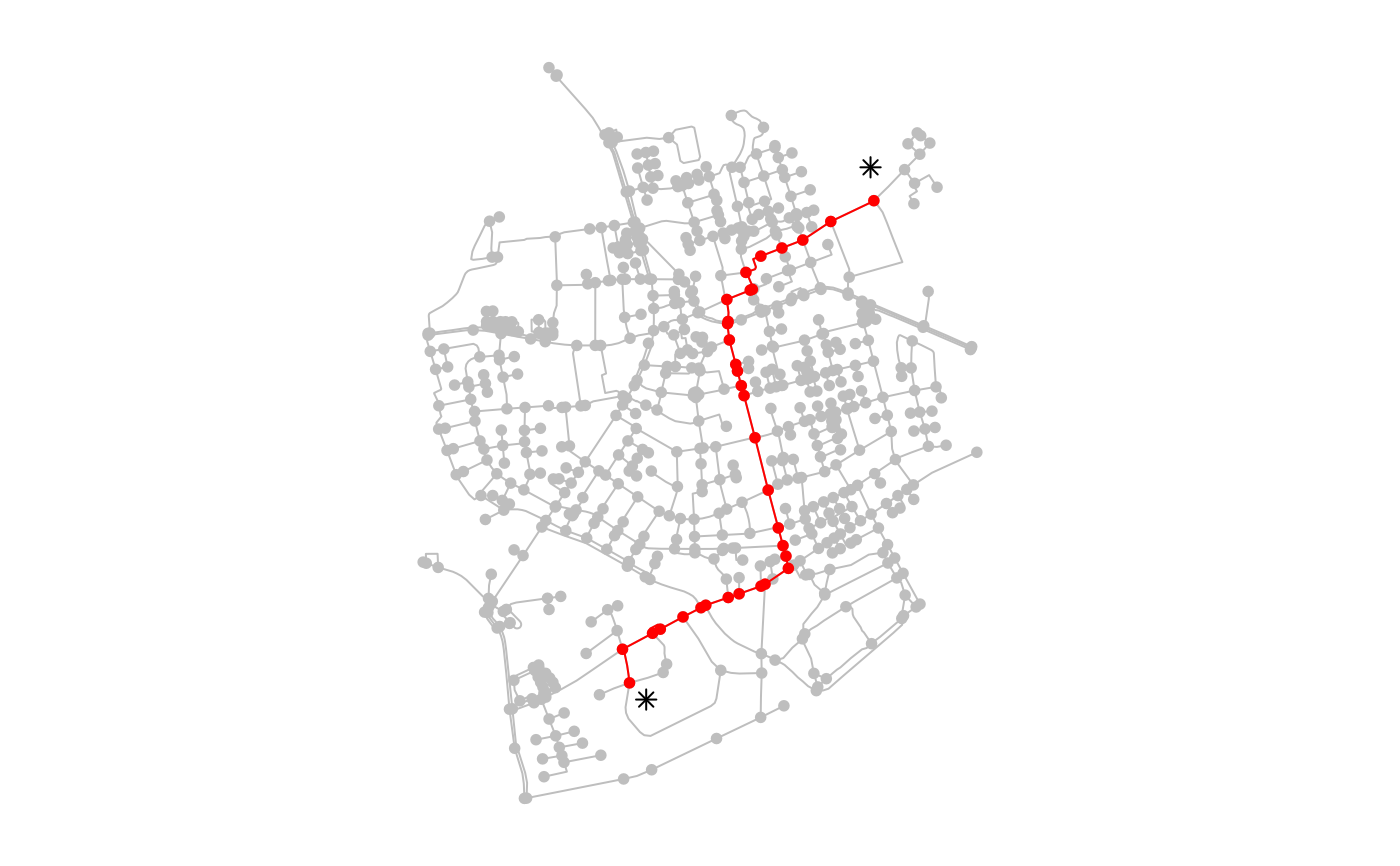Combined wrapper around shortest_paths, all_shortest_paths and all_simple_paths from igraph, allowing to provide any geospatial point as from argument and any set of geospatial points as to argument. If such a geospatial point is not equal to a node in the network, it will be snapped to its nearest node before calculating the shortest or simple paths.

st_network_paths(
x,
from,
to = igraph::V(x),
weights = NULL,
type = "shortest",
use_names = TRUE,
...
)

## Arguments

x

An object of class sfnetwork.

from

The geospatial point from which the paths will be calculated. Can be an object an object of class sf or sfc, containing a single feature. When multiple features are given, only the first one is used. Alternatively, it can be an integer, referring to the index of the node from which the paths will be calculated, or a character, referring to the name of the node from which the paths will be calculated.

to

The (set of) geospatial point(s) to which the paths will be calculated. Can be an object of class sf or sfc. Alternatively it can be a numeric vector containing the indices of the nodes to which the paths will be calculated, or a character vector containing the names of the nodes to which the paths will be calculated. By default, all nodes in the network are included.

weights

The edge weights to be used in the shortest path calculation. Can be a numeric vector giving edge weights, or a column name referring to an attribute column in the edges table containing those weights. If set to NULL, the values of a column named weight in the edges table will be used automatically, as long as this column is present. If not, the geographic edge lengths will be calculated internally and used as weights. If set to NA, no weights are used, even if the edges have a weight column. Ignored when type = 'all_simple'.

type

Character defining which type of path calculation should be performed. If set to 'shortest' paths are calculated using shortest_paths, if set to 'all_shortest' paths are calculated using all_shortest_paths, if set to 'all_simple' paths are calculated using all_simple_paths. Defaults to 'shortest'.

use_names

If a column named name is present in the nodes table, should these names be used to encode the nodes in a path, instead of the node indices? Defaults to TRUE. Ignored when the nodes table does not have a column named name.

...

Arguments passed on to the corresponding igraph or igraph function. Arguments predecessors and inbound.edges are ignored.

## Value

An object of class tbl_df with one row per returned path. Depending on the setting of the type argument, columns can be node_paths (a list column with for each path the ordered indices of nodes present in that path) and edge_paths

(a list column with for each path the ordered indices of edges present in that path). 'all_shortest' and 'all_simple' return only node_paths, while 'shortest' returns both.

## Details

Spatial features provided to the from and/or to argument don't necessarily have to be points. Internally, the nearest node to each feature is found by calling st_nearest_feature, so any feature with a geometry type that is accepted by that function can be provided as from and/or to argument.

When directly providing integer node indices or character node names to the from and/or to argument, keep the following in mind. A node index should correspond to a row-number of the nodes table of the network. A node name should correspond to a value of a column in the nodes table named name. This column should contain character values without duplicates.

For more details on the wrapped functions from igraph see the shortest_paths or all_simple_paths documentation pages.

st_network_cost

## Examples

library(sf, quietly = TRUE)
library(tidygraph, quietly = TRUE)

# Create a network with edge lengths as weights.
# These weights will be used automatically in shortest paths calculation.
net = as_sfnetwork(roxel, directed = FALSE) %>%
st_transform(3035) %>%
activate("edges") %>%
mutate(weight = edge_length())

# Providing node indices.
paths = st_network_paths(net, from = 495, to = 121)
paths
#> # A tibble: 1 × 2
#>   node_paths edge_paths
#>   <list>     <list>
#> 1 <int > <int >

node_path = paths %>%
slice(1) %>%
pull(node_paths) %>%
unlist()
node_path
#>   495 485 244 402 166  18  19  96 299 283   9 167 292 524 111 506 512 657 533
#>  424 426 208 164 260 471 143 136 135 140 478  28  29 121

par(mar = c(1,1,1,1))
plot(net, col = "grey")
plot(slice(activate(net, "nodes"), node_path), col = "red", add = TRUE)par(oldpar)

# Providing nodes as spatial points.
# Points that don't equal a node will be snapped to their nearest node.
p1 = st_geometry(net, "nodes") + st_sfc(st_point(c(50, -50)))
st_crs(p1) = st_crs(net)
p2 = st_geometry(net, "nodes") + st_sfc(st_point(c(-10, 100)))
st_crs(p2) = st_crs(net)

paths = st_network_paths(net, from = p1, to = p2)
paths
#> # A tibble: 1 × 2
#>   node_paths edge_paths
#>   <list>     <list>
#> 1 <int > <int >

node_path = paths %>%
slice(1) %>%
pull(node_paths) %>%
unlist()
node_path
#>   495 485 244 402 166  18  19  96 299 283   9 167 292 524 111 506 512 657 533
#>  424 426 208 164 260 471 143 136 135 140 478  28  29 121

par(mar = c(1,1,1,1))
plot(net, col = "grey")
plot(c(p1, p2), col = "black", pch = 8, add = TRUE)
plot(slice(activate(net, "nodes"), node_path), col = "red", add = TRUE)par(oldpar)

# Using another column for weights.
net %>%
activate("edges") %>%
mutate(foo = runif(n(), min = 0, max = 1)) %>%
st_network_paths(p1, p2, weights = "foo")
#> # A tibble: 1 × 2
#>   node_paths edge_paths
#>   <list>     <list>
#> 1 <int > <int >

# Obtaining all simple paths between two nodes.
# Beware, this function can take long when:
# --> Providing a lot of 'to' nodes.
# --> The network is large and dense.
net = as_sfnetwork(roxel, directed = TRUE)
st_network_paths(net, from = 1, to = 12, type = "all_simple")
#> # A tibble: 6 × 1
#>   node_paths
#>   <list>
#> 1 <int >
#> 2 <int >
#> 3 <int >
#> 4 <int >
#> 5 <int >
#> 6 <int >

# Obtaining all shortest paths between two nodes.
# Not using edge weights.
# Hence, a shortest path is the paths with the least number of edges.
st_network_paths(net, from = 5, to = 1, weights = NA, type = "all_shortest")
#> # A tibble: 2 × 1
#>   node_paths
#>   <list>
#> 1 <int >
#> 2 <int >